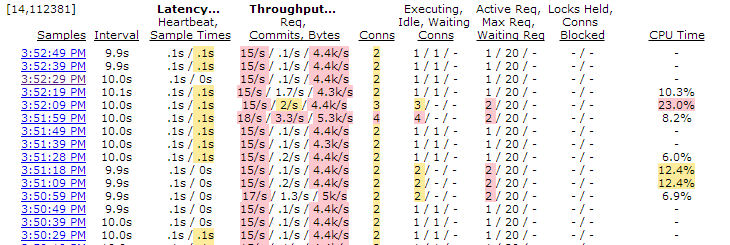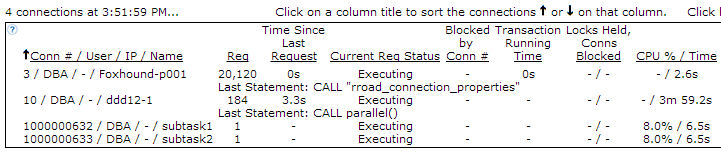## Wednesday, December 5, 2012

### Intra-Procedure Parallelism

Question: How can I make a SQL Anywhere stored procedure run faster?

Answer: One way is to make use of the excess CPU capacity found on many computers by spreading the work across two or more CPUs... not by relying on intra-query parallelism which works on individual SQL statements, but by moving blocks of code out of a single stored procedure into separate events so they run in parallel on separate internal connections.

The title calls this technique "Intra-Procedure Parallelism" but it's really an example of "inter-query parallelism" which is descibed in the Help as "executing different requests simultaneously on separate CPUs"... in this case, the original code that executed as a single request (one procedure call) is changed into separate requests (event invocations) that execute simultaneously. Inter-query parallelism has been around forever in SQL Anywhere, and this article shows how it can be exploited to improve the performance of a single user connection.
Here's an example, a procedure called "serial" that performs two time-consuming steps one after the other:
```CREATE TABLE t (
result_number   INTEGER NOT NULL PRIMARY KEY,
result          BIGINT NOT NULL );

CREATE PROCEDURE serial()
BEGIN

DECLARE @start        TIMESTAMP;
DECLARE @start_step_1 TIMESTAMP;
DECLARE @start_step_2 TIMESTAMP;

-- Step 1.

SET @start        = CURRENT TIMESTAMP;
SET @start_step_1 = CURRENT TIMESTAMP;

INSERT t
ON EXISTING UPDATE
SELECT 1,
SUM ( CAST ( a.row_num AS BIGINT ) )
FROM RowGenerator AS a
CROSS JOIN RowGenerator AS b
CROSS JOIN RowGenerator AS c;
COMMIT;

MESSAGE STRING ( CAST ( DATEDIFF ( MILLISECOND, @start_step_1, CURRENT TIMESTAMP )
AS DECIMAL ( 11, 2 ) ) / 1000.0,
' seconds to perform step 1' ) TO CONSOLE;

-- Step 2.

SET @start_step_2 = CURRENT TIMESTAMP;

INSERT t
ON EXISTING UPDATE
SELECT 2,
SUM ( CAST ( a.row_num AS BIGINT ) )
FROM RowGenerator AS a
CROSS JOIN RowGenerator AS b
CROSS JOIN RowGenerator AS c
CROSS JOIN RowGenerator AS d
WHERE d.row_num <= 2;
COMMIT;

MESSAGE STRING ( CAST ( DATEDIFF ( MILLISECOND, @start_step_2, CURRENT TIMESTAMP )
AS DECIMAL ( 11, 2 ) ) / 1000.0,
' seconds to perform step 2' ) TO CONSOLE;

MESSAGE STRING ( CAST ( DATEDIFF ( MILLISECOND, @start, CURRENT TIMESTAMP )
AS DECIMAL ( 11, 2 ) ) / 1000.0,
' seconds to perform both steps' ) TO CONSOLE;

END;

CALL serial();

10.1680000 seconds to perform step 1
19.9700000 seconds to perform step 2
30.1440000 seconds to perform both steps
```
Both steps ran on the same CPU, ignoring all other processors that might be sitting idle, and together they took a total of 30 seconds to run.

Here's the alternative, a procedure called "parallel" that uses the TRIGGER EVENT statement to launch two events, "subtask1" and "subtasks2":

• The code on lines 14 through 21 initializes subtask_in_progress with a "not yet started" row for each subtask; the CONNECTION_PROPERTY ( 'Number' ) function is used to fill in the task_connection_number, and subtask_connection_number is set to zero.

• The TRIGGER EVENT statements on lines 25 and 26 take almost zero time to execute because they are "fire and forget" operations, not "call and return".

• The code on lines 28 through 37 waits for all the subtask events to get underway, then the code on lines 39 through 57 waits until they are all complete.

• It is important to note that the subtask events (shown later) fill in subtask_in_progress.subtask_connection_number values, but only the caller sets those values back to zero (lines 48 through 55) when they no longer match actual connections. Stuff happens; the subtask events can terminate abnormally, so they can't be relied upon to tell the caller when they're done.
```CREATE TABLE subtask_in_progress (
subtask_number              INTEGER NOT NULL, -- 1, 2

CREATE PROCEDURE parallel()
BEGIN

DECLARE @start TIMESTAMP;

SET @start = CURRENT TIMESTAMP;

-- Initialize subtasks as "not yet started".

WHERE task_connection_number = CONNECTION_PROPERTY ( 'Number' );

INSERT subtask_in_progress VALUES ( CONNECTION_PROPERTY ( 'Number' ), 1, 0 );
INSERT subtask_in_progress VALUES ( CONNECTION_PROPERTY ( 'Number' ), 2, 0 );
COMMIT;

-- Wait for all the subtasks to start.

WHILE EXISTS ( SELECT *
WHERE task_connection_number = CONNECTION_PROPERTY ( 'Number' )
AND subtask_connection_number = 0 ) LOOP

WAITFOR DELAY '00:00:00.5';

END LOOP;

-- Wait for all the subtasks to finish.

WHILE EXISTS ( SELECT *
WHERE task_connection_number = CONNECTION_PROPERTY ( 'Number' )
AND subtask_connection_number <> 0 ) LOOP

WAITFOR DELAY '00:00:00.5';

-- Update any of the subtasks that have finished.

WHERE task_connection_number = CONNECTION_PROPERTY ( 'Number' )
AND CONNECTION_PROPERTY ( 'Number', subtask_connection_number ) IS NULL;
COMMIT;

END LOOP;

MESSAGE STRING ( CAST ( DATEDIFF ( MILLISECOND, @start, CURRENT TIMESTAMP )
AS DECIMAL ( 11, 2 ) ) / 1000.0,
' seconds to perform both tasks' ) TO CONSOLE;

END;

CALL parallel();

21.8280000 seconds to perform both tasks
```
Each subtask event does take longer than corresponding step in the serial solution, but the whole process is a lot faster: 22 seconds instead of 30.

Here's the code for the two subtask events:

• The CREATE EVENT statements on lines 1 and 33 show how to create "untyped" or "user-defined" events; i.e., events that have neither TYPE nor SCHEDULE clauses and can thus be fired only by TRIGGER EVENT statements.

• Lines 8 through 14 and 40 through 46 show how the rows in subtask_in_progress are updated; EVENT_PARAMETER ( 'ConnectionID' ) provides the caller's connection number, and CONNECTION_PROPERTY ( 'Number' ) gives the event's connection number (developers from The Old School might prefer to use @@SPID).

• Lines 16 through 25 is the code from step 1 in the serial solution, and 48 through 59 is the code from step 2.
```CREATE EVENT subtask1
HANDLER BEGIN

DECLARE @start TIMESTAMP;

SET @start = CURRENT TIMESTAMP;

SET subtask_connection_number = CONNECTION_PROPERTY ( 'Number' )
WHERE task_connection_number = EVENT_PARAMETER ( 'ConnectionID' )
COMMIT;

-- Do step 1.

INSERT t
ON EXISTING UPDATE
SELECT 1,
SUM ( CAST ( a.row_num AS BIGINT ) )
FROM RowGenerator AS a
CROSS JOIN RowGenerator AS b
CROSS JOIN RowGenerator AS c;
COMMIT;

MESSAGE STRING ( CAST ( DATEDIFF ( MILLISECOND, @start, CURRENT TIMESTAMP )
AS DECIMAL ( 11, 2 ) ) / 1000.0,
' seconds to perform subtask1' ) TO CONSOLE;

END;

HANDLER BEGIN

DECLARE @start TIMESTAMP;

SET @start = CURRENT TIMESTAMP;

SET subtask_connection_number = CONNECTION_PROPERTY ( 'Number' )
WHERE task_connection_number = EVENT_PARAMETER ( 'ConnectionID' )
COMMIT;

-- Do step 2.

INSERT t
ON EXISTING UPDATE
SELECT 2,
SUM ( CAST ( a.row_num AS BIGINT ) )
FROM RowGenerator AS a
CROSS JOIN RowGenerator AS b
CROSS JOIN RowGenerator AS c
CROSS JOIN RowGenerator AS d
WHERE d.row_num <= 2;
COMMIT;

MESSAGE STRING ( CAST ( DATEDIFF ( MILLISECOND, @start, CURRENT TIMESTAMP )
AS DECIMAL ( 11, 2 ) ) / 1000.0,
' seconds to perform subtask2' ) TO CONSOLE;

END;
```
The CPU usage for the serial and parallel solutions shows how using 2 processors instead of just one can reduce the overall elapsed time from 30 seconds to 22:
Serial CPU UsageParallel CPU UsageHere's another look at the two tests using Foxhound, with the serial test appearing below (earlier in time) than the parallel test:
Serial and Parallel TestsHere's what the connections look like during the serial and parallel tests:
Serial ConnectionParallel ConnectionsIn this example it wasn't actually necessary to create two subtask events, one would have sufficed: the subtask1 event could have handled step 1 and the calling procedure could have performed step 2 and then checked to make sure subtask1 was finished.

As coded, there are three connections in use, two doing actual work (subtask1 and subtask1) and one leaning on a shovel... :)

#### 1 comment:

Anonymous said...

So Foxhound goes MapReduce?

Volker